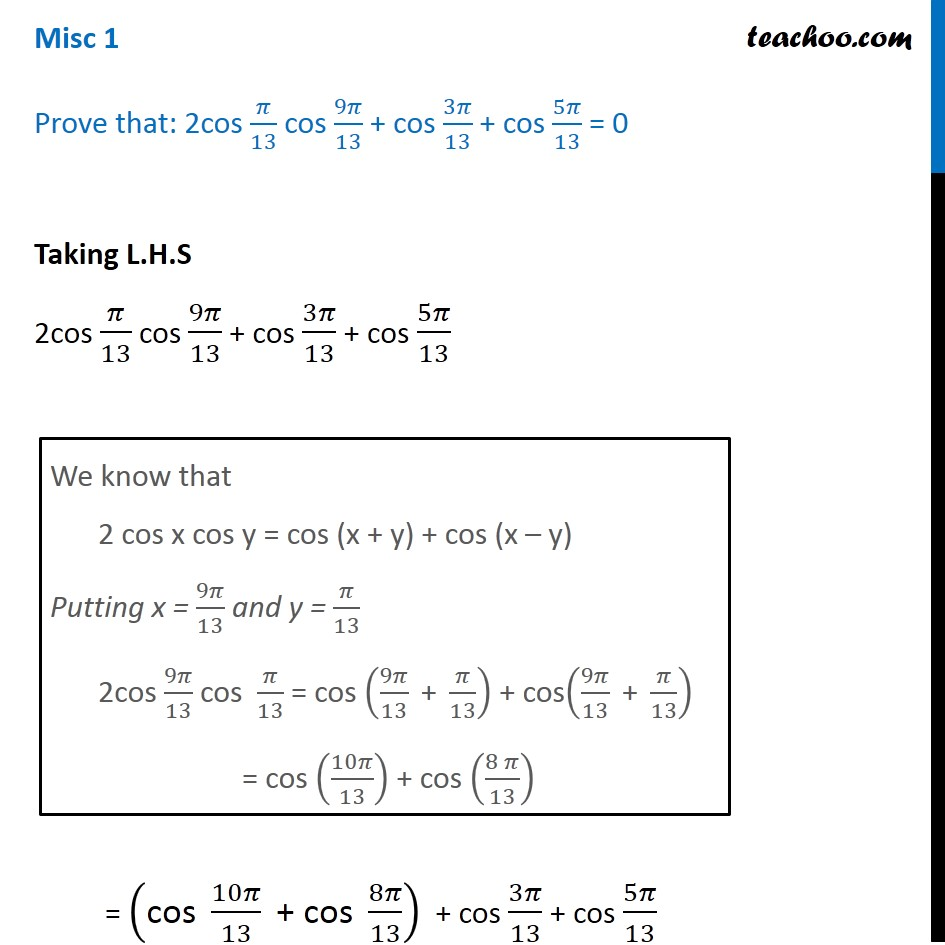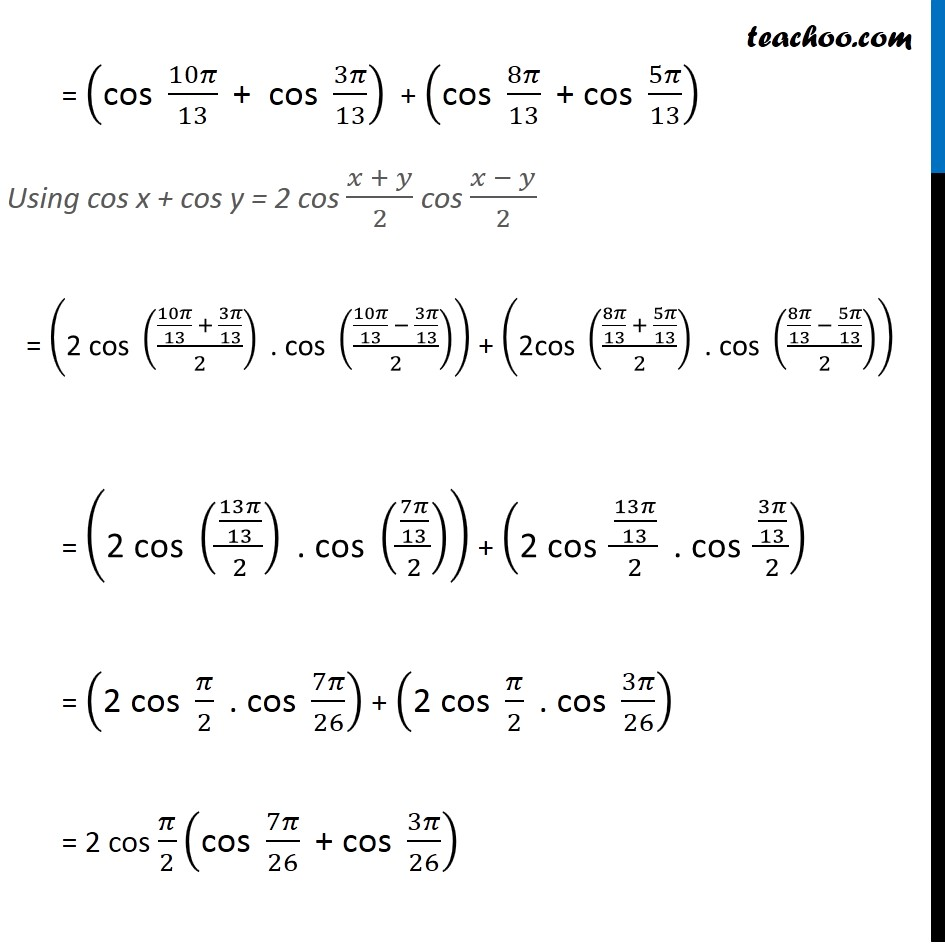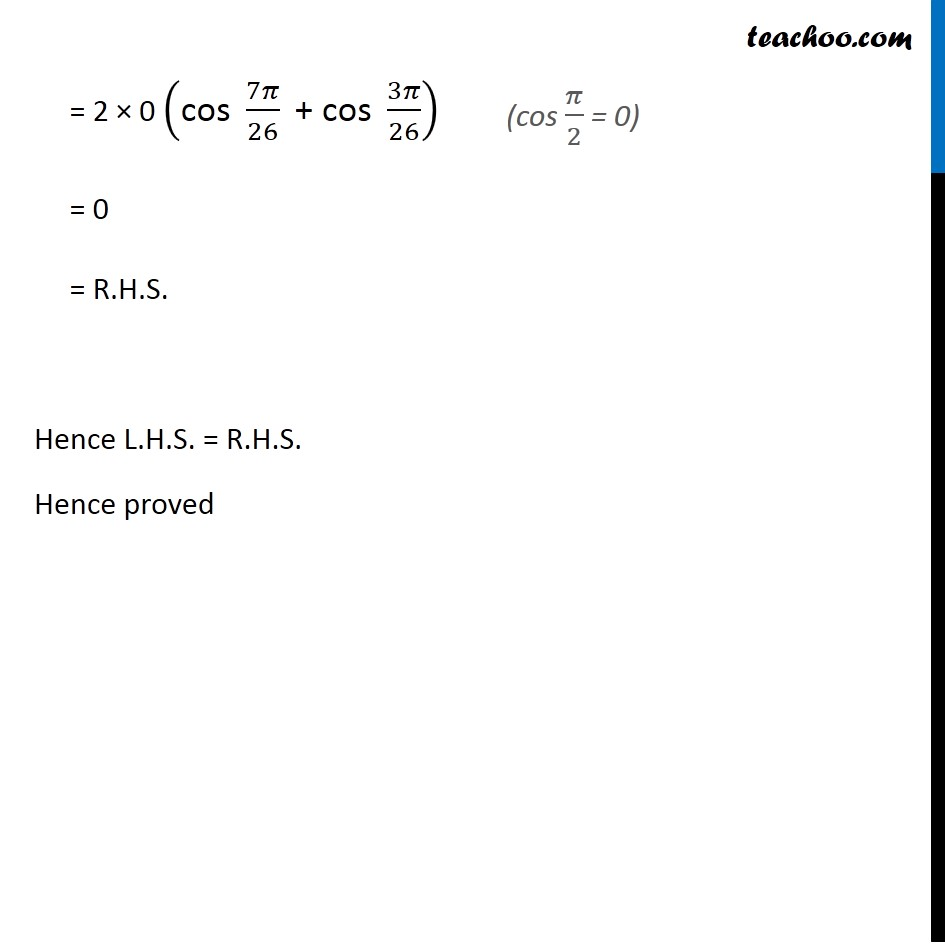Learn All Concepts of Chapter 2 Class 11 Relations and Function - FREE. Check - Trigonometry Class 11 - All Concepts1. Chapter 3 Class 11 Trigonometric Functions
2. Serial order wise
3. Miscellaneous

Transcript

Misc 1 Prove that: 2cos 𝜋/13 cos 9𝜋/13 + cos 3𝜋/13 + cos 5𝜋/13 = 0 Taking L.H.S 2cos 𝜋/13 cos 9𝜋/13 + cos 3𝜋/13 + cos 5𝜋/13 = ("cos " 10𝜋/13 " + cos " 8𝜋/13) + cos 3𝜋/13 + cos 5𝜋/13 We know that 2 cos x cos y = cos (x + y) + cos (x – y) Putting x = 9𝜋/13 and y = 𝜋/13 2cos 9𝜋/13 cos 𝜋/13 = cos (9𝜋/13 " + " 𝜋/13) + cos(9𝜋/13 " + " 𝜋/13) = cos (10𝜋/13) + cos ((8 𝜋)/13) = ("cos " 10𝜋/13 " + cos " 3𝜋/13) + ("cos " 8𝜋/13 " + cos " 5𝜋/13) = ("2 cos " ((10𝜋/13 + 3𝜋/13)/2)" . cos " ((10𝜋/13 − 3𝜋/13)/2)) + ("2cos " ((8𝜋/13 + 5𝜋/13)/2)" . cos " ((8𝜋/13 − 5𝜋/13)/2)) = ("2 cos " ((13𝜋/13)/2)" . cos " ((7𝜋/13)/2)) + ("2 cos " (13𝜋/13)/2 " . cos " (3𝜋/13)/2) = ("2 cos " 𝜋/2 " . cos " 7𝜋/26) + ("2 cos " 𝜋/2 " . cos " 3𝜋/26) = 2 cos 𝜋/2 ("cos " 7𝜋/26 " + cos " 3𝜋/26) = 2 × 0 ("cos " 7𝜋/26 " + cos " 3𝜋/26) = 0 = R.H.S. Hence L.H.S. = R.H.S. Hence proved (cos 𝜋/2 = 0)

Miscellaneous